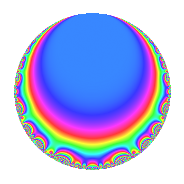# Properties

 Label 283.1.b.bLevel 283 Weight 1 Character orbit 283.b Analytic conductor 0.141 Analytic rank 0 Dimension 2 Projective image $$S_{4}$$ CM/RM No Inner twists 2

# Related objects

## Newspace parameters

 Level: $$N$$ = $$283$$ Weight: $$k$$ = $$1$$ Character orbit: $$[\chi]$$ = 283.b (of order $$2$$ and degree $$1$$)

## Newform invariants

 Self dual: No Analytic conductor: $$0.141235398575$$ Analytic rank: $$0$$ Dimension: $$2$$ Coefficient field: $$\Q(\sqrt{-2})$$ Coefficient ring: $$\Z[a_1, a_2]$$ Coefficient ring index: $$1$$ Projective image $$S_{4}$$ Projective field Galois closure of 4.2.283.1 Artin image size $$48$$ Artin image $\GL(2,3)$ Artin field Galois closure of 8.2.22665187.3

## $q$-expansion

Coefficients of the $$q$$-expansion are expressed in terms of $$\beta = \sqrt{-2}$$. We also show the integral $$q$$-expansion of the trace form.

 $$f(q)$$ $$=$$ $$q -\beta q^{2} + \beta q^{3} - q^{4} + \beta q^{5} + 2 q^{6} - q^{7} - q^{9} +O(q^{10})$$ $$q -\beta q^{2} + \beta q^{3} - q^{4} + \beta q^{5} + 2 q^{6} - q^{7} - q^{9} + 2 q^{10} + q^{11} -\beta q^{12} + q^{13} + \beta q^{14} -2 q^{15} - q^{16} + \beta q^{18} -\beta q^{19} -\beta q^{20} -\beta q^{21} -\beta q^{22} - q^{23} - q^{25} -\beta q^{26} + q^{28} - q^{29} + 2 \beta q^{30} -\beta q^{31} + \beta q^{32} + \beta q^{33} -\beta q^{35} + q^{36} -2 q^{38} + \beta q^{39} + q^{41} -2 q^{42} -\beta q^{43} - q^{44} -\beta q^{45} + \beta q^{46} + \beta q^{47} -\beta q^{48} + \beta q^{50} - q^{52} + \beta q^{55} + 2 q^{57} + \beta q^{58} + q^{59} + 2 q^{60} + q^{61} -2 q^{62} + q^{63} + q^{64} + \beta q^{65} + 2 q^{66} -\beta q^{69} -2 q^{70} -\beta q^{75} + \beta q^{76} - q^{77} + 2 q^{78} -\beta q^{80} - q^{81} -\beta q^{82} -2 q^{83} + \beta q^{84} -2 q^{86} -\beta q^{87} - q^{89} -2 q^{90} - q^{91} + q^{92} + 2 q^{93} + 2 q^{94} + 2 q^{95} -2 q^{96} + q^{97} - q^{99} +O(q^{100})$$ $$\operatorname{Tr}(f)(q)$$ $$=$$ $$2q - 2q^{4} + 4q^{6} - 2q^{7} - 2q^{9} + O(q^{10})$$ $$2q - 2q^{4} + 4q^{6} - 2q^{7} - 2q^{9} + 4q^{10} + 2q^{11} + 2q^{13} - 4q^{15} - 2q^{16} - 2q^{23} - 2q^{25} + 2q^{28} - 2q^{29} + 2q^{36} - 4q^{38} + 2q^{41} - 4q^{42} - 2q^{44} - 2q^{52} + 4q^{57} + 2q^{59} + 4q^{60} + 2q^{61} - 4q^{62} + 2q^{63} + 2q^{64} + 4q^{66} - 4q^{70} - 2q^{77} + 4q^{78} - 2q^{81} - 4q^{83} - 4q^{86} - 2q^{89} - 4q^{90} - 2q^{91} + 2q^{92} + 4q^{93} + 4q^{94} + 4q^{95} - 4q^{96} + 2q^{97} - 2q^{99} + O(q^{100})$$

## Character Values

We give the values of $$\chi$$ on generators for $$\left(\mathbb{Z}/283\mathbb{Z}\right)^\times$$.

 $$n$$ $$3$$ $$\chi(n)$$ $$-1$$

## Embeddings

For each embedding $$\iota_m$$ of the coefficient field, the values $$\iota_m(a_n)$$ are shown below.

For more information on an embedded modular form you can click on its label.

Label $$\iota_m(\nu)$$ $$a_{2}$$ $$a_{3}$$ $$a_{4}$$ $$a_{5}$$ $$a_{6}$$ $$a_{7}$$ $$a_{8}$$ $$a_{9}$$ $$a_{10}$$
282.1
 1.41421i − 1.41421i
1.41421i 1.41421i −1.00000 1.41421i 2.00000 −1.00000 0 −1.00000 2.00000
282.2 1.41421i 1.41421i −1.00000 1.41421i 2.00000 −1.00000 0 −1.00000 2.00000
 $$n$$: e.g. 2-40 or 990-1000 Significant digits: Format: Complex embeddings Normalized embeddings Satake parameters Satake angles

## Inner twists

Char. orbit Parity Mult. Self Twist Proved
1.a Even 1 trivial yes
283.b Odd 1 yes

## Hecke kernels

This newform can be constructed as the kernel of the linear operator $$T_{2}^{2} + 2$$ acting on $$S_{1}^{\mathrm{new}}(283, [\chi])$$.